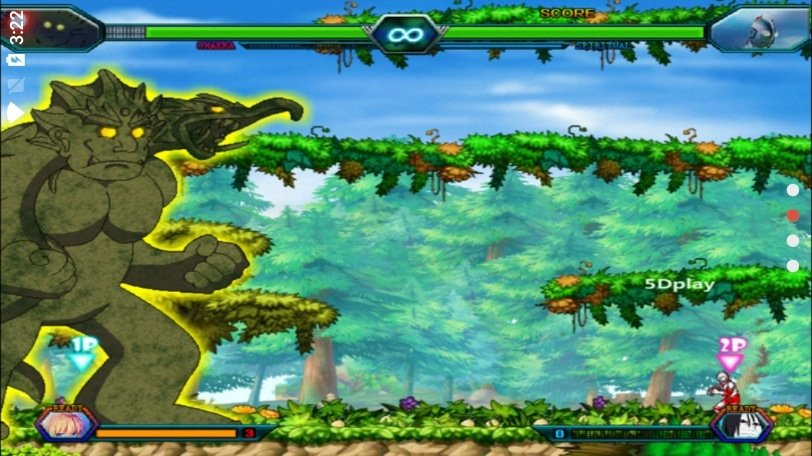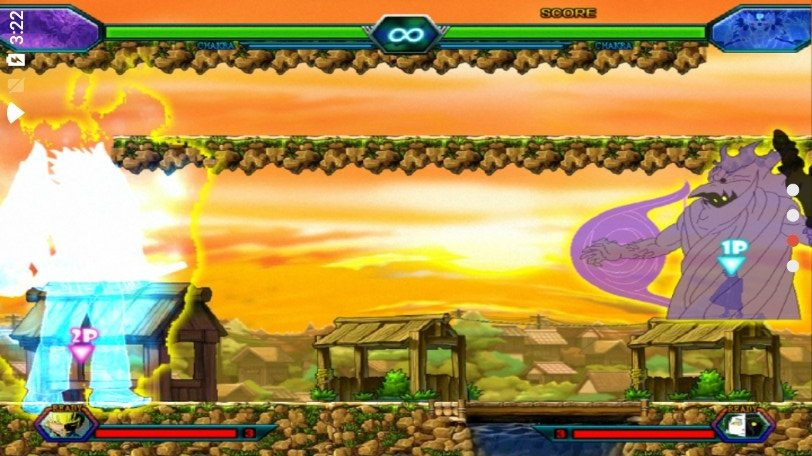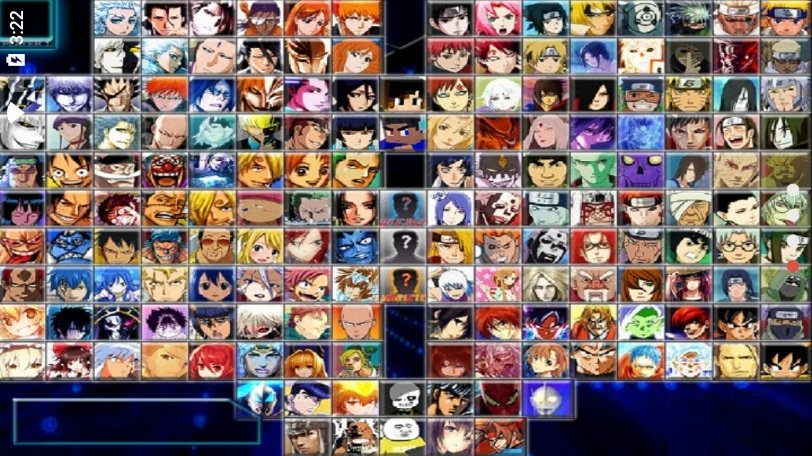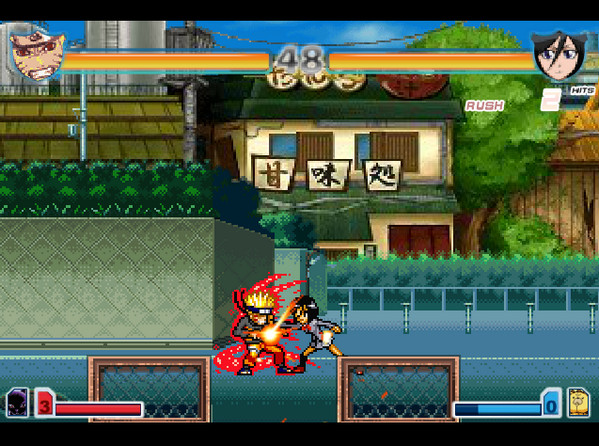## 摸摸鱼死神vs火影## 游戏介绍

### 死神vs火影出招表：

1.黑崎一护： J+K 卍解

2.漩涡鸣人： J+K 九尾化

3.朽木 露琪亚：J 踢一下 J+J 二连击 J+J+J 三连击 K 跳一下 K+K 大跳 K+J 跳起来踢一下 W+J 向斜上踢 S+J 闪到身后打飞敌人 W+U 向斜上的波 S+U 暴一下? I 小招(费一格气) W+I 同上(费一格气) S+I 变死神(费三格气)

4春野 樱：J 打一下 J+J 二连击 J+J+J 三连击 K 跳一下 K+K 大跳 K+J 跳起来踢一下 W+J 倒立+踢 S+J 扔石头 W+U 向斜上的苦无 S+U 向下打一拳 I 小招(费一格气) W+I 同上(费一格气) S+I 小樱的内心(费三格气)

5.日番谷 冬狮郎：J 砍一下 J+J 二连击 J+J+J 三连击 K 跳一下 K+K 大跳 K+J 跳起突袭 W+J 突袭 S+J 暴气 W+U 向斜上砍 S+U 向外暴气 I 冰龙(费一格气) W+I 冰柱(费一格气) S+I 大招(费三格气)

6.宇智波 佐助：J 打一拳 J+J 三连击 J+J+J 四连击 K 跳一下 K+K 大跳 K+J 跳起踢 W+J 草剃剑四连击 S+J 草剃剑突袭 W+U 踢上去 S+U 草剃剑向上砍 I 千鸟(费一格气) W+I 千鸟流(费一格气) S+I草剃剑连击+豪火球(费三格气)VS电脑：和电脑进行对战，可以锻炼自己的角色操作能力。

### 摸摸鱼死神vs火影评价：